#### 1.   Plot the graph y = 2x2 + 10x + 8 when x is −6, −3 and 0. There are also the coordinates (−5, 8), (−4, 0), (−2, −4), (−1, 0) and (1, 20)

 (a) Using a table work out the values of y

y = 2x2 + 10x + 8

 x −6 −3 0 x2 36 9 0 2x2 72 18 0 + 10x −60 −30 0 + 8 + 8 + 8 + 8 y 20 −4 8

 (b) The coordinates of the above are (−6, 20), (−5, 8), (−4, 0), (−3, −4), (−2, −4), (−1, 0), (0, 8) and (1, 20)

 (c) Plot the above coordinates and draw a line through these points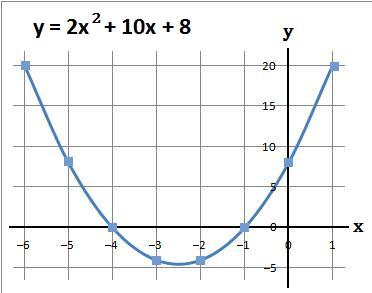#### 2.   Plot the graph y = 3x2 + 21x + 18 when x = −8, −4 and 0. There are also the coordinates (−6, 0) and (−2, −12)

 (a) Using a table work out the values of y

y = 3x2 + 21x + 18

 x −8 −4 0 x2 64 16 0 3x2 192 48 0 + 21x −168 −84 0 + 18 + 18 + 18 + 18 y 42 −18 18

 (b) The coordinates of the above are (−8, 42), (−6, 0), (−4, −18), (−2, −12) and (0, 18)

 (c) Plot the above coordinates and draw a line through these points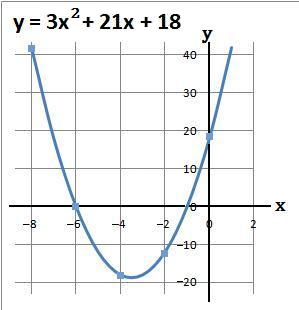#### 3.   Plot the graph y = 4x2 + 20x − 24 when x = −6, −2 and 2. There are also the coordinates (−4, −40) and (0, −24)

 (a) Using a table work out the values of y

y = 4x2 + 20x − 24

 x −6 −2 2 x2 36 4 4 4x2 144 16 16 + 20x −120 −40 40 − 24 − 24 − 24 − 24 y 0 −48 32

 (b) The coordinates of the above are (−6, 0), (−4, −40), (−2, −48), (0, −24) and (2, 32)

 (c) Plot the above coordinates and draw a line through these points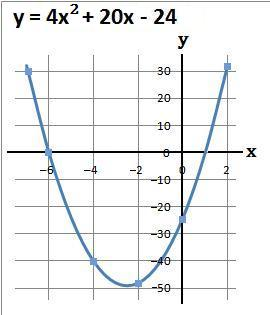#### 4.   Plot the graph y = 2x2 + 9x − 56 when x = −8, −2 and 4. There are also the coordinates (−6, −38), (−4, −60), (0, −56) and (2, −30)

 (a) Using a table work out the values of y

y = 2x2 + 9x − 56

 x −8 −2 4 x2 64 4 16 2x2 128 8 32 + 9x −72 −18 36 − 56 − 56 − 56 − 56 y 0 −66 12

 (b) The coordinates of the above are (−8, 0), (−6, −38), (−4, −60), (−2, −66), (0, −56), (2, −30) and (4, 12)

 (c) Plot the above coordinates and draw a line through these points#### 5.   Plot the graph y = 5x2 − 20x − 60 when x = −4, 2 and 8. There are also the coordinates (−2, 0), (0, −60), (4, −60) and (6, 0)

 (a) Using a table work out the values of y

y = 5x2 − 20x − 60

 x −4 2 8 x2 16 4 64 5x2 80 20 320 − 20x +80 −40 −160 − 60 − 60 − 60 − 60 y 100 −80 100

 (b) The coordinates of the above are (−4, 100), (−2, 0), (0, −60), (2, −80), (4, −60), (6, 0) and (8, 100)

 (c) Plot the above coordinates and draw a line through these points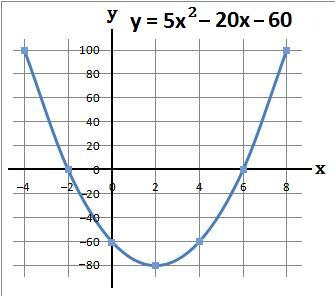#### 6.   Plot the graph y = 3x2 − 21x + 36 when x = 0, 3 and 6. There are also the coordinates (1, 18), (2, 6), (4, 0), (5, 6) and (7, 36)

 (a) Using a table work out the values of y

y = 3x2 − 21x + 36

 x 0 3 6 x2 0 9 36 3x2 0 27 108 − 21x 0 −63 −126 + 36 + 36 + 36 + 36 y 36 0 18

 (b) The coordinates of the above are (0, 36), (1, 18), (2, 6), (3, 0), (4, 0), (5, 6), (6, 18) and (7, 36)

 (c) Plot the above coordinates and draw a line through these points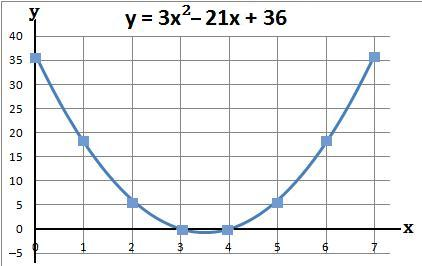#### 7.   Plot the graph y = 3x2 − 40x + 48 when x = 0, 6 and 12. There are also the coordinates (2, −20), (4, −64), (8, −80), (10, −52) and (14, 76)

 (a) Using a table work out the values of y

y = 3x2 − 40x + 48

 x 0 6 12 x2 0 36 144 3x2 0 108 432 − 40x 0 −240 −480 + 48 + 48 + 48 + 48 y 48 −84 0

 (b) The coordinates of the above are (0, 48), (2, −20), (4, −64), (6, −84), (8, −80), (10, −52), (12, 0) and (14, 76)

 (c) Plot the above coordinates and draw a line through these points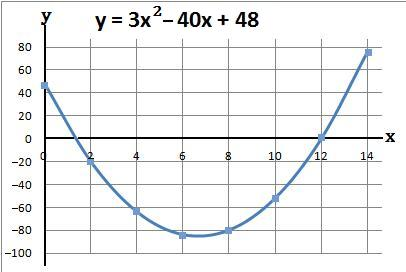back to: